Download Presentation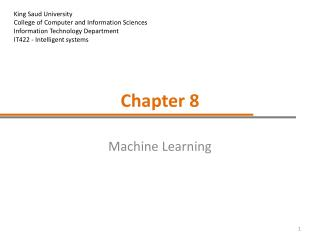Chapter 8

# Chapter 8 - PowerPoint PPT Presentation

King Saud University College of Computer and Information Sciences Information Technology Department IT422 - Intelligent systems. Chapter 8. Machine Learning. Introduction. What is learning? Learning in humans consists of (at least): memorization, comprehension, learning from examples.I am the owner, or an agent authorized to act on behalf of the owner, of the copyrighted work described.
Download Presentation## Chapter 8

An Image/Link below is provided (as is) to download presentation

Download Policy: Content on the Website is provided to you AS IS for your information and personal use and may not be sold / licensed / shared on other websites without getting consent from its author.While downloading, if for some reason you are not able to download a presentation, the publisher may have deleted the file from their server.

- - - - - - - - - - - - - - - - - - - - - - - - - - E N D - - - - - - - - - - - - - - - - - - - - - - - - - -
Presentation Transcript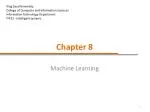King Saud University

College of Computer and Information Sciences

Information Technology Department

IT422 - Intelligent systems

### Chapter 8

Machine Learning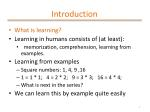Introduction
• What is learning?
• Learning in humans consists of (at least):
• memorization, comprehension, learning from examples.
• Learning from examples
• Square numbers: 1, 4, 9 ,16
• 1 = 1 * 1; 4 = 2 * 2; 9 = 3 * 3; 16 = 4 * 4;
• What is next in the series?
• We can learn this by example quite easily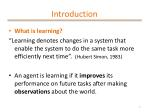Introduction
• What is learning?

“Learning denotes changes in a system that enable the system to do the same task more efficiently next time”. (Hubert Simon, 1983)

• An agent is learning if it improves its performance on future tasks after making observations about the world.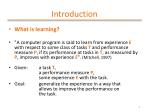Introduction
• What is learning?
• "A computer program is said to learn from experience E with respect to some class of tasks T and performance measure P, if its performance at tasks in T, as measured by P, improves with experience E". (Mitchell, 1997)
• Given: a task T, a performance measure P, some experience E with the task.
• Goal: generalize the experience in a way that allows to improve the performance on the task.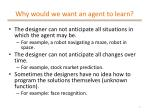Why would we want an agent to learn?
• The designer can not anticipate all situations in which the agent may be.
• For example, a robot navigating a maze, robot in space.
• The designer can not anticipate all changes over time.
• For example, stock market prediction.
• Sometimes the designers have no idea how to program the solutions themselves (unknown function).
• For example: face recognition.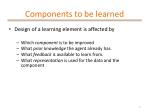Components to be learned
• Design of a learning element is affected by
• Which component is to be improved
• What prior knowledge the agent already has.
• What feedback is available to learn from.
• What representation is used for the data and the component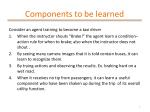Components to be learned

Consider an agent training to become a taxi driver

• When the instructor shouts “Brake!” the agent learn a condition–action rule for when to brake; also when the instructor does not shout .
• By seeing many camera images that it is told contain buses, it can learn to recognize them.
• By trying actions and observing the results. Ex. braking hard on a wet road.
• When it receives no tip from passengers, it can learn a useful component who have been shaken up during the trip of its overall utility function.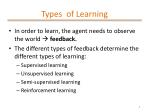Types of Learning
• In order to learn, the agent needs to observe the world  feedback.
• The different types of feedback determine the different types of learning:
• Supervised learning
• Unsupervised learning
• Semi-supervised learning
• Reinforcement learning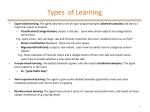Types of Learning
• Supervised learning: The agent observes a set of input-output examples (labeled examples) and learns a map from inputs to outputs.
• Classification (Categorization): output is discrete . Learn why certain objects are categorized a certain way.

E.g.: spam email, why are dogs, cats and humans mammals, but trout, mackerel and tuna are fish?

• Binary classification (Boolean): there are only two values.
• Regression(Prediction): output is real-valued . Learn how to predict how to categorize unseen objects

E.g., Given examples of financial stocks and a categorization of them into safe and unsafe stocks

Learn how to predict whether a new stock will be safe.

• Unsupervised learning: No explicit feedback is given, only the inputs (unlabeled examples). The agent learns patterns in the input.
• Ex. “good traffic days”
• Semi-supervised learning: The agent is given some labeled examples (generally a few) and some unlabeled examples and tries to learn a mapping.
• Reinforcement learning: The agent learns from a series of rewards and punishments, and based on these adapts its behavior (e.g. playing chess) .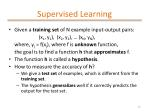Supervised Learning
• Given a training set of N example input-output pairs:

(x1, y1), (x2, y2), … (xN, yN),

where, yj = f(xj), where f is unknown function,

the goal is to find a function h that approximates f.

• The function h is called a hypothesis.
• How to measure the accuracy of h?
• We give a test set of examples, which is different from the training set.
• The hypothesis generalizes well if it correctly predicts the output for the test set.How to select a hypothesis

(a)

(b)

First, select the hypothesis space: in this case, the set of polynomials.

(a): The line is consistent with the data.

(b): The high-degree polynomial is also consistent with the data.

Ockham’s razor: Choose the simplest hypothesis which is consistent with the data.Decision Trees
• A decision tree represents a function that has multiple inputs but a single output a “decision”.
• We focus on discrete input and Boolean output (Boolean classification)
• A decision tree reaches the decision by a set of tests on the attributes (the inputs). Thus, the internal nodes are the tests and the leaf nodes are the decisions.
• Example:

Decision nodes

Test nodesDecision Trees
• A more complex example: deciding to wait at a restaurant:
• The attributes :
• Alternate: whether there is a suitable alternative restaurant nearby.
• Bar: whether the restaurant has a comfortable bar area to wait in.
• Fri I Sat: true on Fridays and Saturdays.
• Hungry: whether we are hungry.
• Patrons: how many people are in the restaurant (values are None, Some, and Full).
• Price: the restaurant's price range (\$, \$\$, \$\$\$).
• Raining: whether it is raining outside.
• Reservation: whether we made a reservation.
• Type: the kind of restaurant (French, Italian, Thai, or burger).
• WaitEstimate: the wait estimated by the host (0-10 minutes, 10-30, 30-60, or >60).Decision Trees
• Classification of examples is positive (T) or negative (F)Decision Trees
• This is the real function.
• Our goal is to learn this function from examples.Decision Trees
• A decision tree can be expressed as propositional logic sentence (Boolean function) in DNF (disjunctive normal form):
• Goal  (Path1 V Path2 V … Pathn), where Pathi= (Attribute1 = Valuek1  Attribute2 = Valuek2 …)
• The same Boolean function can have many representations as a decision tree (just change the order of the attributes)  We want the smallest possible tree:
• Example: The decision tree of P  (Q  R)Decision Trees

The order of the attributes: Q, R,P

The order of the attributes: P, Q, R

Smaller number of nodes  The order is important

A decision tree for the function: P  (Q  R).Decision Trees
• For n (Boolean) attributes there are 2^(2^n) different Boolean functions, and the number of decision trees is much larger (more than n! 2^(2^n) )
• Example: n = 6, there are approximately 18.4 x 10^18 possible Boolean functions
• Exhaustive search is impossible in practice  Learning the decision tree  greedy heuristic search
• How to choose the most important attribute and build the decision tree?
• Several algorithms exist. Ex. ID3 (Iterative Dichotomiser 3)Summary
• Learning takes many forms, depending on the nature of the agent, the component to be improved, and the available feedback.
• Learning can be supervised, unsupervised, semi-supervised learning, and reinforcement learning, depending on the given feedback.
• Decision trees are powerful tools for classification, they can represent rules in tree structure where each node is either test or decision node.